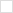TWiki>P1076 Web>Vhdl202X_MeetingMinutes>2020_0827_Meeting (2020-08-27, JimLewis)

# P1076 Minutes from August 27, 2020

## Attendees

• Peter Flake, Unai Martinez-Corral, Lieven, Jim

## Meeting Discussion

• Issues of VHDL-2008 aggregates wrt LHS?
• Goal:

```(Cout, Sum8) <= ('0' & A8) & B8 ;

-- All OK:
type slv_2d is array (natural range <>) of std_logic_vector;

constant aggregate1  : std_logic_vector := ('1', "1010", '0');
constant aggregate2  : std_logic_vector := ('1', ('1', ('0', ('1', '0'))));
constant aggregate22 : std_logic_vector := ('1', ('1', ('0', "10")));
constant aggregate3  : std_logic_vector := (((('1', '1'), '0'), '1'), '0');
constant aggregate4  : slv_2d           := ((((('1'), '1'), '0'), '1'), ('0', '1', '0', '1'));
```
-- Problematic with GHDL:
```type my_new_type is array(natural range <>) of std_logic_vector(31 downto 0);

-- Then I declare a constant of that new type
constant constant_of_my_new_type : my_new_type (0 to 7) := ((others=>(others => '0')));

signal signal_of_my_new_type 		: my_new_type (0 to 7);

begin

process(clk_i)
begin

-- if Reset low then signal_of_my_new_type = constant_of_my_new_type
-- else, signal_of_my_new_type is filled with one at the next clock rising edge

if n_rst_i = '0' then
signal_of_my_new_type <= constant_of_my_new_type;
elsif rising_edge(clk_i) then
signal_of_my_new_type <= ((others=>(others => '1')));
end if;```

## Next Meeting

10 September 2020, 11 am Pacific Daylight Time (GMT-7)

## Comments

Topic revision: r1 - 2020-08-27 - 19:48:02 - JimLewis

Copyright © 2008-2021 by the contributing authors. All material on this collaboration platform is the property of the contributing authors.
Ideas, requests, problems regarding TWiki? Send feedback